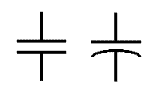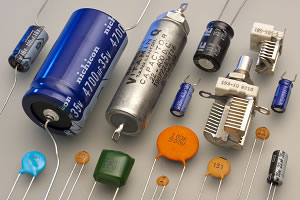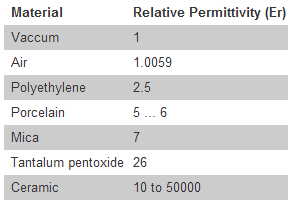# What is a Capacitor?

Capacitors are a electrical / electronic device made of two metal plates separated by an insulator or dielectric. A dielectric or an insulator is a material that prevents the flow of the electric current from one plate to the other.

Capacitors stores energy in the form of an electric field (it is clear when the capacitors works with direct current) and the capacitance is the amount of electric charges that it is capable to store.Capacitor SymbolsCapacitors” by Eric Schrader from San Francisco, CA, USA – 12739s. Licensed under CC BY-SA 2.0 via Wikimedia Commons.

Capacitance depends on the physical characteristics of the capacitor.

• If the area of the plates, that are face to face, is large the capacitance increases.
• If the gap between plates increases, the capacitance decreases.
• The type of dielectric material between the plates also affects the capacitance.
• If you increase the applied voltage, the stored charge increases.

## What is the dielectric material used for?

The dielectric material is used to increase the capacitance of the capacitor. Different materials are used as dielectric. These materials have different degrees of permittivity. (different capacities to create an electric field)The greater the permittivity, the greater the capacitance. The capacitance is given by the formula: C = Er x A/d, where:

• C = capacitance
• Er = permittivity
• A = area between plates
• D = separation between the plates

The unit of measurement is the Farad. There are submultiples such as: miliFarad (mF), microFarad (UF), nanoFarad (nF) and picoFarad (pF). The main electrical features of the capacitor are its capacitance and the maximum voltage between the plates (maximum voltage that can withstand the capacitor without damage).

Never connect a capacitor to a higher voltage that it can withstand
since it can explode.

•
•
•
•
•
•
•
•
•
•
•
•
•
•
•
•